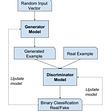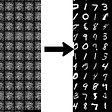# Generating Cifar-10 Fake Images using Deep Convolutional Generative Adversarial Networks (DCGAN)

So guys in today’s blog we will see how can we build some real-looking fake images, using Deep Convolutional Generative Adversarial Networks or DCGANs. GANs are basically known for their two networks, Generative network, and Discriminative network.

We train our Discriminative model in such a way that it can tell us that which image is real and which image is fake. The generative network tries to create new images that can even fool the Discriminator network and prove themselves to be real. So without any further due.

# Let’s do it…

## Code for Deep Convolutional Generative Adversarial Networks (DCGAN)…

`# Deep Convolutional GANs# Importing the librariesfrom __future__ import print_functionimport torchimport torch.nn as nnimport torch.nn.parallelimport torch.optim as optimimport torch.utils.dataimport torchvision.datasets as dsetimport torchvision.transforms as transformsimport torchvision.utils as vutilsfrom torch.autograd import Variable# Setting some hyperparametersbatchSize = 64 # We set the size of the batch.imageSize = 64 # We set the size of the generated images (64x64).# Creating the transformationstransform = transforms.Compose([transforms.Scale(imageSize), transforms.ToTensor(), transforms.Normalize((0.5, 0.5, 0.5), (0.5, 0.5, 0.5)),]) # We create a list of transformations (scaling, tensor conversion, normalization) to apply to the input images.# Loading the datasetdataset = dset.CIFAR10(root = './data', download = True, transform = transform) # We download the training set in the ./data folder and we apply the previous transformations on each image.dataloader = torch.utils.data.DataLoader(dataset, batch_size = batchSize, shuffle = True, num_workers = 2) # We use dataLoader to get the images of the training set batch by batch.# Defining the weights_init function that takes as input a neural network m and that will initialize all its weights.def weights_init(m):    classname = m.__class__.__name__    if classname.find('Conv') != -1:        m.weight.data.normal_(0.0, 0.02)    elif classname.find('BatchNorm') != -1:        m.weight.data.normal_(1.0, 0.02)        m.bias.data.fill_(0)# Defining the generatorclass G(nn.Module): # We introduce a class to define the generator.    def __init__(self): # We introduce the __init__() function that will define the architecture of the generator.        super(G, self).__init__() # We inherit from the nn.Module tools.        self.main = nn.Sequential( # We create a meta module of a neural network that will contain a sequence of modules (convolutions, full connections, etc.).            nn.ConvTranspose2d(100, 512, 4, 1, 0, bias = False), # We start with an inversed convolution.            nn.BatchNorm2d(512), # We normalize all the features along the dimension of the batch.            nn.ReLU(True), # We apply a ReLU rectification to break the linearity.            nn.ConvTranspose2d(512, 256, 4, 2, 1, bias = False), # We add another inversed convolution.            nn.BatchNorm2d(256), # We normalize again.            nn.ReLU(True), # We apply another ReLU.            nn.ConvTranspose2d(256, 128, 4, 2, 1, bias = False), # We add another inversed convolution.            nn.BatchNorm2d(128), # We normalize again.            nn.ReLU(True), # We apply another ReLU.            nn.ConvTranspose2d(128, 64, 4, 2, 1, bias = False), # We add another inversed convolution.            nn.BatchNorm2d(64), # We normalize again.            nn.ReLU(True), # We apply another ReLU.            nn.ConvTranspose2d(64, 3, 4, 2, 1, bias = False), # We add another inversed convolution.            nn.Tanh() # We apply a Tanh rectification to break the linearity and stay between -1 and +1.        )    def forward(self, input): # We define the forward function that takes as argument an input that will be fed to the neural network, and that will return the output containing the generated images.        output = self.main(input) # We forward propagate the signal through the whole neural network of the generator defined by self.main.        return output # We return the output containing the generated images.# Creating the generatornetG = G() # We create the generator object.netG.apply(weights_init) # We initialize all the weights of its neural network.# Defining the discriminatorclass D(nn.Module): # We introduce a class to define the discriminator.    def __init__(self): # We introduce the __init__() function that will define the architecture of the discriminator.        super(D, self).__init__() # We inherit from the nn.Module tools.        self.main = nn.Sequential( # We create a meta module of a neural network that will contain a sequence of modules (convolutions, full connections, etc.).            nn.Conv2d(3, 64, 4, 2, 1, bias = False), # We start with a convolution.            nn.LeakyReLU(0.2, inplace = True), # We apply a LeakyReLU.            nn.Conv2d(64, 128, 4, 2, 1, bias = False), # We add another convolution.            nn.BatchNorm2d(128), # We normalize all the features along the dimension of the batch.            nn.LeakyReLU(0.2, inplace = True), # We apply another LeakyReLU.            nn.Conv2d(128, 256, 4, 2, 1, bias = False), # We add another convolution.            nn.BatchNorm2d(256), # We normalize again.            nn.LeakyReLU(0.2, inplace = True), # We apply another LeakyReLU.            nn.Conv2d(256, 512, 4, 2, 1, bias = False), # We add another convolution.            nn.BatchNorm2d(512), # We normalize again.            nn.LeakyReLU(0.2, inplace = True), # We apply another LeakyReLU.            nn.Conv2d(512, 1, 4, 1, 0, bias = False), # We add another convolution.            nn.Sigmoid() # We apply a Sigmoid rectification to break the linearity and stay between 0 and 1.        )    def forward(self, input): # We define the forward function that takes as argument an input that will be fed to the neural network, and that will return the output which will be a value between 0 and 1.        output = self.main(input) # We forward propagate the signal through the whole neural network of the discriminator defined by self.main.        return output.view(-1) # We return the output which will be a value between 0 and 1.# Creating the discriminatornetD = D() # We create the discriminator object.netD.apply(weights_init) # We initialize all the weights of its neural network.# Training the DCGANscriterion = nn.BCELoss() # We create a criterion object that will measure the error between the prediction and the target.optimizerD = optim.Adam(netD.parameters(), lr = 0.0002, betas = (0.5, 0.999)) # We create the optimizer object of the discriminator.optimizerG = optim.Adam(netG.parameters(), lr = 0.0002, betas = (0.5, 0.999)) # We create the optimizer object of the generator.for epoch in range(25): # We iterate over 25 epochs.    for i, data in enumerate(dataloader, 0): # We iterate over the images of the dataset.                # 1st Step: Updating the weights of the neural network of the discriminator        netD.zero_grad() # We initialize to 0 the gradients of the discriminator with respect to the weights.                # Training the discriminator with a real image of the dataset        real, _ = data # We get a real image of the dataset which will be used to train the discriminator.        input = Variable(real) # We wrap it in a variable.        target = Variable(torch.ones(input.size())) # We get the target.        output = netD(input) # We forward propagate this real image into the neural network of the discriminator to get the prediction (a value between 0 and 1).        errD_real = criterion(output, target) # We compute the loss between the predictions (output) and the target (equal to 1).                # Training the discriminator with a fake image generated by the generator        noise = Variable(torch.randn(input.size(), 100, 1, 1)) # We make a random input vector (noise) of the generator.        fake = netG(noise) # We forward propagate this random input vector into the neural network of the generator to get some fake generated images.        target = Variable(torch.zeros(input.size())) # We get the target.        output = netD(fake.detach()) # We forward propagate the fake generated images into the neural network of the discriminator to get the prediction (a value between 0 and 1).        errD_fake = criterion(output, target) # We compute the loss between the prediction (output) and the target (equal to 0).        # Backpropagating the total error        errD = errD_real + errD_fake # We compute the total error of the discriminator.        errD.backward() # We backpropagate the loss error by computing the gradients of the total error with respect to the weights of the discriminator.        optimizerD.step() # We apply the optimizer to update the weights according to how much they are responsible for the loss error of the discriminator.        # 2nd Step: Updating the weights of the neural network of the generator        netG.zero_grad() # We initialize to 0 the gradients of the generator with respect to the weights.        target = Variable(torch.ones(input.size())) # We get the target.        output = netD(fake) # We forward propagate the fake generated images into the neural network of the discriminator to get the prediction (a value between 0 and 1).        errG = criterion(output, target) # We compute the loss between the prediction (output between 0 and 1) and the target (equal to 1).        errG.backward() # We backpropagate the loss error by computing the gradients of the total error with respect to the weights of the generator.        optimizerG.step() # We apply the optimizer to update the weights according to how much they are responsible for the loss error of the generator.                # 3rd Step: Printing the losses and saving the real images and the generated images of the minibatch every 100 steps        print('[%d/%d][%d/%d] Loss_D: %.4f Loss_G: %.4f' % (epoch, 25, i, len(dataloader), errD.data, errG.data)) # We print les losses of the discriminator (Loss_D) and the generator (Loss_G).        if i % 100 == 0: # Every 100 steps:            vutils.save_image(real, '%s/real_samples.png' % "./results", normalize = True) # We save the real images of the minibatch.            fake = netG(noise) # We get our fake generated images.            vutils.save_image(fake.data, '%s/fake_samples_epoch_%03d.png' % ("./results", epoch), normalize = True) # We also save the fake generated images of the minibatch.`

1st epoch:

## 2nd epoch:

3rd epoch:

23rd epoch:

24th epoch:

25th epoch:

Do let me know if there’s any query regarding Deep Convolutional Generative Adversarial Networks (DCGAN) by contacting me on email or LinkedIn.

So this is all for this blog folks, thanks for reading it and I hope you are taking something with you after reading this and till the next time 👋…

Read my previous post: HELMET AND NUMBER PLATE DETECTION AND RECOGNITION USING YOLOV3

--

--

--

## More from MLearning.ai

Data Scientists must think like an artist when finding a solution when creating a piece of code. ⚪️ Artists enjoy working on interesting problems, even if there is no obvious answer ⚪️ linktr.ee/mlearning 🔵 Follow to join our 18K+ Unique DAILY Readers 🟠

## 5 Types of Machine Learning Algorithms You Need to Know## Core Technologies of Alibaba Cloud Voice Recognition Model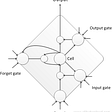## How to Use K-Means Algorithm to Create Customer Segmentation Tools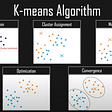## Build a Deep Learning Classifier of Dog Breeds using Keras## Load A Machine Learning Model Inside The Docker Container## Natural Language Processing with Quora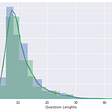## Convolutional Neuronal Network with Keras-Tuner on CIFAR 10## Summaries big text with a seq2seq Network and Attention## Abhishek Sharma

Data Scientist || Blogger || machinelearningprojects.net

## More from Medium